# How to Reverse First Name and Last Name

This post explains that how to flip or reverse the first name and last name in one column. How to reverse the first name and last name in each cell in one column in excel. How to flip the first name and last name with excel formula or excel VBA macro.

If you need to reverse first name and last name in a column and then put the result into another cell in another column.  You need to get or extract the first name or last name firstly and then combine those names into the same cell. You can write an excel formula or write a new excel VBA code to achieve it.

## Reverse First Name and Last Name with excel formula

To reverse first name and last name, you can create a formula based on the MID function, the FIND function and the Len function as follows:

`=MID(B2&" " &B2, FIND(" ",B2)+1, LEN(B2))`

Let’s see how this formula works:

=B2&” ” &B2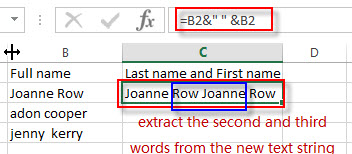This formula will use the concatenate operator to join text string in B2 with text string in B2 again to generate a new text string. Then we just need to extract the second and third words from the new text string.

= FIND(” “,B2)+1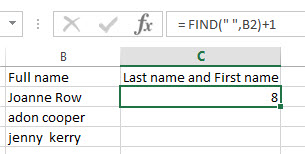This formula will return the position of the first space character in text string of Cell B2.  Then it will pass into the MID function as its start_num argument.

= LEN(B2)

x
How to Show All Named Ranges in Excel

It will return the length of the text string in Cell B2, then it will pass into the MID function as its num_chars argument.

=MID(B2&” ” &B2, FIND(” “,B2)+1, LEN(B2))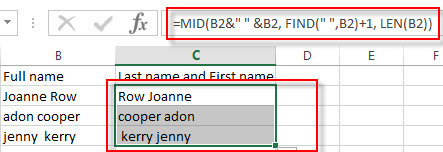## Reverse First Name and Last Name with excel VBA macro

You can also write an excel macro to reverse the first name and last name, you can refer to the following steps:

1# click on “Visual Basic” command under DEVELOPER Tab.2# then the “Visual Basic Editor” window will appear.

3# click “Insert” ->”Module” to create a new module4# paste the below VBA code into the code window. Then clicking “Save” button.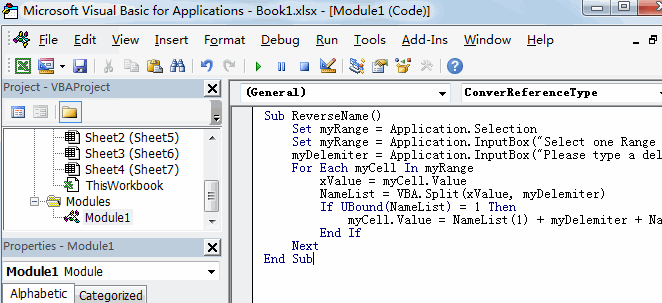```Sub ReverseName()
Set myRange = Application.Selection
Set myRange = Application.InputBox("Select one Range that you want to reverse name", "ReverseName", myRange.Address, Type:=8)
myDelemiter = Application.InputBox("Please type a delimiter character:", "ReverseName", " ", Type:=2)
For Each myCell In myRange
xValue = myCell.Value
NameList = VBA.Split(xValue, myDelemiter)
If UBound(NameList) = 1 Then
myCell.Value = NameList(1) + myDelemiter + NameList(0)
End If
Next
End Sub```

5# back to the current worksheet, then run the above excel macro.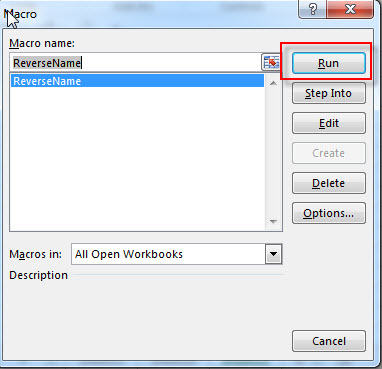6# select the range of cells that contains the first name and last name, then click “OK” button.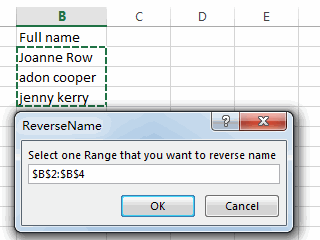7# enter one delemiter character, such as: sapce character in the text box. Then click OK button.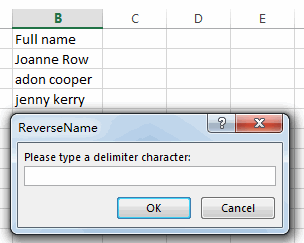8# you will see that the first name and last name have been reversed in the selected range of cells.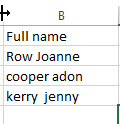### Related Formulas

• Get Last Name From Full Name
To extract the last name within a Full name in excel. You need to use a combination of the RIGHT function, the LEN function, the FIND function and the SUBSTITUTE function to create a complex formula in excel..…
• Get First Name From Full Name
If you want to get the first name from a full name list in Column B, you can use the FIND function within LEFT function in excel. The generic formula is as follows:=LEFT(B1,FIND(” “,B1)-1).…
• Split full name to first and last name
You can create another formula based on the RIGHT function, the LEN function, the FIND function and the SUBSTITUTE function to extract the last name……
• Extract text before first comma or space
If you want to extract text before the first comma or space character in cell B1, you can use a combination of the LEFT function and FIND function….
• Extract nth word from text string
If you want to extract the nth word from a text string in a single cell, you can create an excel formula based on the TRIM function, the MID function, the SUBSTITUTE function, the REPT function and the LEN function..…

### Related Functions

• Excel MID function
The Excel MID function returns a substring from a text string at the position that you specify.The syntax of the MID function is as below:= MID (text, start_num, num_chars)….
• Excel FIND function
The Excel FIND function returns the position of the first text string (sub string) within another text string.The syntax of the FIND function is as below:= FIND(find_text, within_text,[start_num])…
• Excel LEN function
The Excel LEN function returns the length of a text string (the number of characters in a text string).The LEN function is a build-in function in Microsoft Excel and it is categorized as a Text Function.The syntax of the LEN function is as below:= LEN(text)…

Related Posts

VBA Macro For VLOOKUP From Another Sheet

In the previous post, you should know that how to fix or remove the #N/A error when using VLOOKUP formula to lookup value from another sheet. And this post will show you how to use VBA code to vlookup data ...

How To Insert Comments in Protected Worksheet in Excel

This post will show you how to allow comments in a protected worksheet in Excel. You can easily to insert comments into cells in a normal worksheet in Excel, but if want to insert a comment in a worksheet that ...

How To Convert Text to Upper Cases(Using VBA) in Excel

This post will show you how to switch from lower case to upper case in Excel. and I am going to show you two different ways of converting text to upper cases using formula or VBA macro in Excel 2013,Excel ...

How To Hide Every Other Row in Excel (Using VBA)

This post will show you how to hide alternate rows or columns in Excel or how to hide every third, fourth, fifth row or column in Excel. If you want to hide every other row in your current worksheet, how ...

How to Disable the Save As Prompt in Excel

This post will show you how to use a VBA Macro to save an Excel file and overwrite any existing file without a prompt so that you are going to get the little window that says file already exists do ...

How to Count Cells that Contain even or odd numbers in Excel

This post will guide you how to count the number of cells that contain odd or even numbers within a range of cells using a formula in Excel 2013/2016.How do I count cells that contain odd numbers through the use ...

How to Count Cells that Contain negative Numbers in Excel

This post will guide you how to count the number of cells that contain negative numbers within a range of cells using a formula in Excel 2013/2016.You can count the number of negative numbers in your data using easy functions ...

How to Count Cells Are Not Blank or Empty in Excel

This post will guide you how to count cells that are not blank or empty in a given range cells using a formula in Excel 2013/2016.How do I count the number of cells that are not blank in a particular ...

How to Count Cells Not equal to X or Y in Excel

This post will guide you how to count the number of cells not equal to criteria X or Y in a given range cells using a formula in Excel 2013/2016.You can easily to count cells equal to or not equal ...

How to Count Cells Less Than a Specific Value in Excel

This post will guide you how to count the number of cells less than a particular numeric value in a given range cells using a formula in Excel 2013/2016. How do I count cells that are less than a specific ...

Sidebar